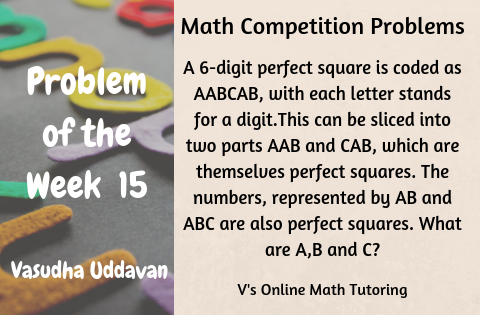top of pageSearch

# Math Competition Problems - Problem of the Week - 15

Updated: May 18, 2019

A 6-digit perfect square is coded as AABCAB, with each letter stands for a digit.This can be sliced into two parts AAB and CAB, which are themselves perfect squares. The numbers, represented by AB and ABC are also perfect squares. What are A,B and C?

- Source QuoraThis week it's not a problem from any of the Math competitions. However, this problem was too interesting to pass. Hence here it is :)

Solution:

First, let's find what AB is. That’s the easiest because it can have only 6 possibilities.

List all the 2 digit squares

16, 25, 36, 49, 64, 81

Now you need to find AAB.

116, 225, 336, 449, 664, 881

Out of these only 225 is a perfect square.

So you know that A=2, B=5

Now to find CAB that’s a perfect square

C cannot be 2 because A=2

Hence out of 125, 325, 425, 525, 625, 725, 825 and 925 only 625 is a perfect square.

And to check ABC which is 256; 256 is also a perfect square!

So your number AABCAB is 225625 which is a perfect square.

So A=2, B=5,C=6Did you like the problem? Would you like to have these problems and solutions delivered to your inbox as I publish them? Click here to subscribe

Previous Week's Problem and Next Week's Problem